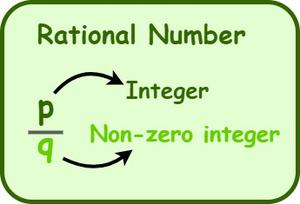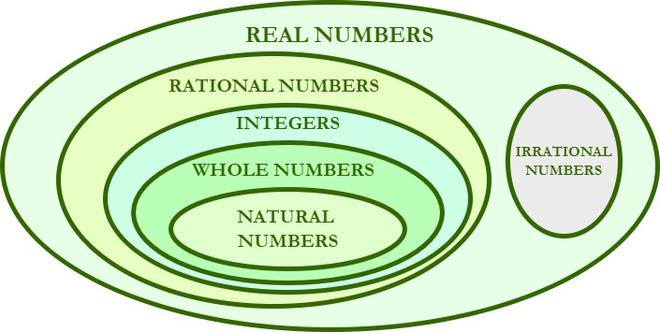GeeksforGeeks App
Open AppBrowser
Continue

# Prove that for all integers a and b, if a ≠ 0, the equation ax + b has a rational solution

In mathematics, a rational number is a type of real number that is in the form of p/q, where p and q are integers and q ≠ 0. The term “rational” is extracted from the word “ratio”. So, any fraction that represents a ratio and that has a non-zero denominator is said to be a rational number. 1/3, 2/5, 3/8, 5/11, etc. are some of the examples of rational numbers. Even “0” is also a rational number, as we can express it in many forms, such as 0/1, 0/2, 0/3, 0/4, and so on. However, fractional numbers with zero in the denominator are irrational, as they give us infinite values. For example, 1/0, 2/0, 3/0, 4/0, etc. are not rational numbers.## Types of Rational Numbers

The letter “Q” stands for rational numbers, which include various types of numbers which include natural numbers, whole numbers, integers, fractions of integers, and decimals (terminating decimals and recurring decimals). Every rational number (Q) is a real number (R), and there are various types of rational numbers as given below.

1. Every integer, such as -7, -2, 0, 5, 8, etc., is a rational number. Integers include both natural numbers and whole numbers.
2. Every fraction whose numerators and denominators are integers, such as -3/7, -2/5, 1/3, 4/9, etc., is a rational number.
3. All terminating decimals, such as 0.28, 0.5321, 0.075, etc., are rational numbers.
4. All non-terminating repeating decimals, such as 0.212121….., 0.111111……, etc., are rational numbers.## How to Identify a Rational Number?

To identify whether a number is rational or not, go through the following characteristics.

1. Natural numbers, whole numbers, integers, fractions of integers, and decimals (terminating decimals and recurring decimals) are all considered to be rational numbers.
2. We can tell that a number is rational if its decimal form is terminating or recurring. For example, 2.8 and 1.424242 are rational numbers.
3. We can tell that a number is irrational if its decimal form is endless or non-recurring. For example, √7 is an irrational number whose value is 2.645751311064591…
4. If a number is represented in the form of p/q, where p and q are integers and q ≠ 0, then the given number is said to be a rational number.

Example: Is 2.7182818284590452……. a rational number?

Solution:

The given number is 2.7182818284590452… It is a non-terminating and non-recurring decimal, so it is not a rational number. Hence, it is an irrational number.

## Prove that for all integers a and b, if a ≠ 0, the equation ax + b has a rational solution

Solution:

Given,

ax + b = 0

Where a, and b are integers and a ≠ 0.

Note: If any number can be expressed in the form of p/q, where p and q are integers and q ≠ 0, then the answer is a rational solution.

ax + b = 0

By adding (−b) on both sides, we get

⇒ ax + b − b = 0 − b

⇒ ax = −b

Now, by multiplying (1/a) on both sides, we get

⇒ ax × (1/a) = −b × (1/a)

⇒ x = −b/a, where a, and b are integers and a ≠ 0.

−b/a is a rational number as it is expressed in the form of p/q, and p and q are integers and q ≠ 0.

Here, a and b are integers, and a ≠ 0. So, −b/a is a rational number.

So, the equation ax + b has a rational solution, for all a, b ∈ Z (integers), a ≠ 0.

Hence proved.

## Solved Examples based on Rational Numbers

Example 1: Identify whether the given numbers are rational or irrational.

(a) 2.56  (b) 0.03  (c) 3.5  (d) √5  (e) 0.75

Solution:

The given numbers are in decimal form. So, to determine whether the given number is rational or not, we have to convert it into the fraction form (i.e., p/q). If the denominator of the fraction obtained is not equal to zero, then the number is rational, otherwise, it is irrational.

a) 2.56

2.56 can be written as 256/100 in a fractional form which is further simplified to 64/25.

So, 2.56 is a rational number.

b) 0.03

0.003 can be written as 3/100 in a fractional form.

So, 0.03 is a rational number.

c) 3.5

3.5 can be written as 35/10 in a fractional form which is further simplified to 7/2.

So, 3.5 is a rational number.

d) √5

The value of √5 is 2.23606797749979…. This is a non-terminating and non recurring value and hence it cannot be written as a fraction. So, √5 is an irrational number.

e) 0.75

0.75 can be written as 75/100 in a fractional form which is further simplified to 3/4.

So, 0.75 is a rational number.

Example 2: Identify whether √5 is a rational number or not.

Solution:

The given number is √5. The value of √5 is 2.23606797749979… It is a non-terminating and non-recurring decimal, i.e., it is not a rational number. Therefore, √5  is an irrational number.

Example 3: Identify whether 3¼ is a rational number or not.

Solution:

The given number is 3¼, which is a mixed fraction.

Its simplest form is ((3 × 4) + 1)/4 = 13/4

The numerator of 13/4 is 13, which is an integer, and its denominator is 4, which is also an integer, and it is not equal to zero.

Hence, 13/4, i.e., 3¼ is a rational number.

## FAQs on Rational Numbers

Question 1: What do we mean by rational numbers explain with examples.

Numbers that are written in the form of  p/q, where p and q are integers, and q does not equal 0 are called rational numbers. Few examples of rational numbers are 2/3, -5/1, 11/7, etc.

Question 2: Write the difference between rational and irrational numbers.

Rational numbers can be expressed in the form of p/q where p and q are integers, eg 2, 3/2, etc, whereas irrational numbers cannot be expressed in form of p/q like, π, e, etc are irrational numbers.

Question 3: Can 0 be considered a rational number?

Yes, 0 can be considered a rational number because it can be written in form such as 0/1 which is comparable to p/q where q is a non-zero integer.

Question 4: Can 3.14 be considered a rational number?